# Continuity#### Everything You Need in One Place

Homework problems? Exam preparation? Trying to grasp a concept or just brushing up the basics? Our extensive help & practice library have got you covered.#### Learn and Practice With Ease

Our proven video lessons ease you through problems quickly, and you get tonnes of friendly practice on questions that trip students up on tests and finals.#### Instant and Unlimited Help

Our personalized learning platform enables you to instantly find the exact walkthrough to your specific type of question. Activate unlimited help now!Get the most by viewing this topic in your current grade. Pick your course now.

##### Intros
###### Lessons
1. Introduction to Continuity
2. Discuss CONTINUITY in everyday language: a function whose graph has no break in it.
3. Discuss CONTINUITY in the context of Calculus:
A function $f$ is continuous at a number a, if: $\lim_{x \to a^-} f(x) = \lim_{x \to a^+} f(x) = f(a)$

Classify different types of discontinuities: removable VS. infinite VS. jump

Analyze: rational function with a hole: $f(x)=\frac{(x-1)(x-2)}{(x-2)}$
VS.
rational function with an asymptote: $g(x)=\frac{1}{x+1}$
VS.
piecewise function: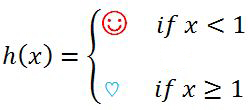4. Lesson Overview - Continuity
##### Examples
###### Lessons
1. Discussing "Continuity" Graphically
The graph of a function $f$ is shown below.
1. State the numbers at which $f$ is discontinuous.
2. Explain and classify each discontinuity.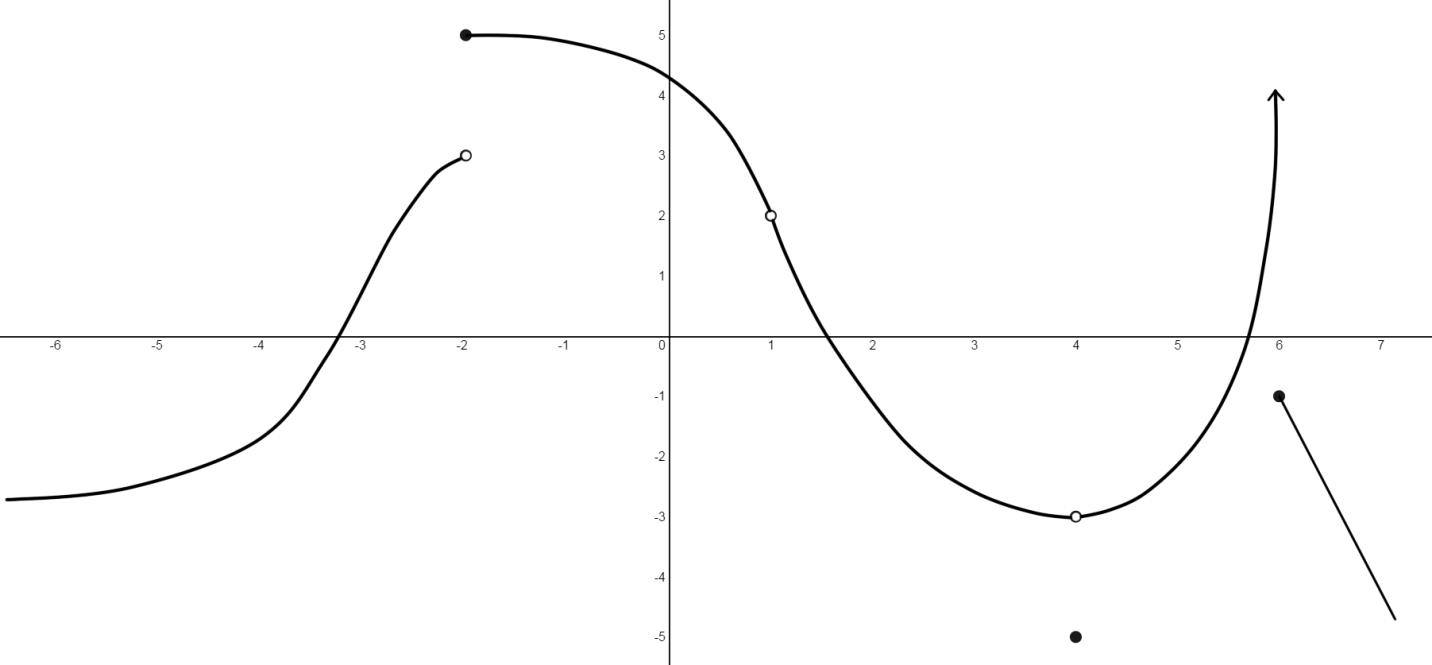2. Detecting Discontinuities Are the following functions continuous at $x=3$?
i) $f(x)=\frac{x^2-2x-3}{x-3}$

ii)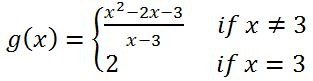iii)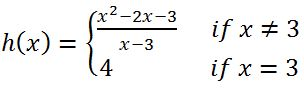1. Discontinuities of Rational Functions (denominator=0)
Locate and classify each discontinuity of the function: $f(x)=\frac{x^2-9}{x^2+x-6}$
1. Discussing "Continuity" Algebraically
Find the values of a and b that make the function $f$ continuous on ($-\infty$, $\infty$).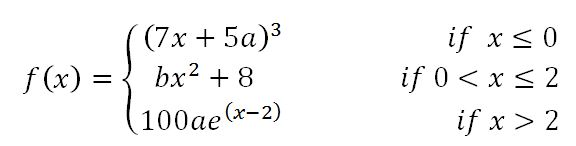###### Free to Join!
StudyPug is a learning help platform covering math and science from grade 4 all the way to second year university. Our video tutorials, unlimited practice problems, and step-by-step explanations provide you or your child with all the help you need to master concepts. On top of that, it's fun - with achievements, customizable avatars, and awards to keep you motivated.
• #### Easily See Your ProgressWe track the progress you've made on a topic so you know what you've done. From the course view you can easily see what topics have what and the progress you've made on them. Fill the rings to completely master that section or mouse over the icon to see more details.
• #### Make Use of Our Learning Aids###### Practice Accuracy

Get quick access to the topic you're currently learning.

See how well your practice sessions are going over time.

Stay on track with our daily recommendations.

• #### Earn Achievements as You LearnMake the most of your time as you use StudyPug to help you achieve your goals. Earn fun little badges the more you watch, practice, and use our service.
• #### Create and Customize Your AvatarPlay with our fun little avatar builder to create and customize your own avatar on StudyPug. Choose your face, eye colour, hair colour and style, and background. Unlock more options the more you use StudyPug.
###### Topic Notes
The idea of continuity lies in many things we experience in our daily lives, for instance, the time it takes you to log into StudyPug and read this section. Limits and continuity are so related that we cannot only learn about one and ignore the other. We will learn about the relationship between these two concepts in this section.
• Definition of "continuity" in everyday language
A function is continuous if it has no holes, asymptotes, or breaks. A continuous graph can be drawn without removing your pen from the paper.

• Definition of "continuity" in Calculus
A function $f$ is continuous at a number a, if: $\lim_{x \to a^-} f(x) = \lim_{x \to a^+} f(x) = f(a)$

• Polynomials are always continuous everywhere. Rational functions are continuous wherever the functions are defined; in other words, avoiding holes and asymptotes, rational functions are continuous everywhere. A function f is continuous at a number a, if and only if:
$\lim_{x \to a^-} f(x) = \lim_{x \to a^+} f(x) = f(a)$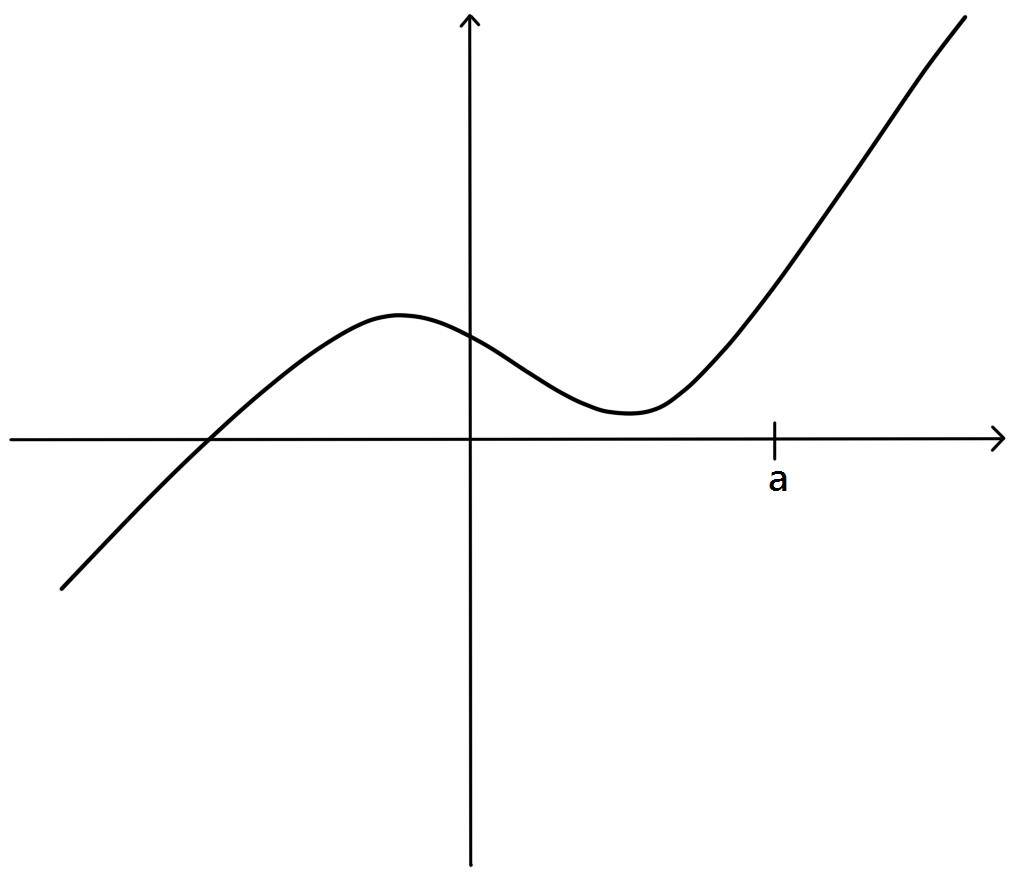In simple words, the graph of a continuous function has no break in it and can be drawn without lifting your pen from the paper.